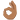# Damped Thermal Oscillations

• A
Hello,

I am attempting to solve the 1 d heat equation using separation of variables.

1d heat equation:

##\frac{\partial T}{\partial t} = \alpha \frac{\partial^2 T}{\partial x^2}##

I used the standard separation of variables to get a solution. Without including boundary conditions right now, the solutions are:

##T(x,t) = f(x) \times g(t)##

where

##f(x) = A_1(sin(\sqrt{\lambda} x) + cos(\sqrt{\lambda} x))## and
##g(t) = A_1 e^{-\lambda \alpha t}##

other terms:
##\alpha =## thermal conductivity
##\lambda =## some constant (solved for in boundary conditions)
##A_1 =## some constant

I've worked through and have a decent grasp on many of the fixed boundary conditions solutions for this problem. I'm trying to model ground temperature with increasing depth, so I know need to have a periodic boundary condition at the surface to represent seasons and then constant temperature deep underground.

Boundary conditions:

##T(0,t) = T_0 + Asin(\frac{2 \pi t}{365} )## (ground surface)

##T(\infty, t) = T_0 ##

##\lim_{x \to \infty} \frac{d T}{d x} = 0 ##

This paper gave the magnitude of thermal oscillations as decreasing in proportion to ##e^\frac{-x}{d_p}## where ##d_p = \sqrt{\alpha t_p/\pi}## is the period depth, t_p is the period time (365 days). I'm not sure how he got that decaying exponential, some kind of coefficient, but I haven't dealt with periodic boundary conditions before and it looks like he didn't use numerical methods to get this one?

Any idea how he was able to calculate the dampening of oscillations??

mjc123
Homework Helper
Try T = T0 + Aei(ωt-βx)e-αx

mjc123
Homework Helper
Oh, and it's "damping". "Dampening" means making moist.

I've been playing with that solution for the past couple of days, a couple insights:
• The method of separation of variables as described here wont work. The solution you provided has an x component in the imaginary exponential term as well as a time component, so it's not two independent functions multiplied.
• The general form looks good although I know it can't be a diffusivity term on the last part, the units need to work out to be dimensionless. I guess according to Eskilson it's ##\frac{1}{\sqrt{2 \alpha / \omega}}##, just not sure how he got that.
I see now how it's required to get that beta term in the sinusoidal function, it accounts for the fact that heat lags behind the wave on the surface. Do you know of any literature I can look into regarding heat functions like this?

Also thank you for the grammatical correction. =)

Nidum
@Nidum looks like that'll be a lot of fun. Thanks, appreciate it.